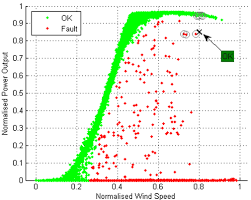### Introduction

k-Nearest Neighbours (k-NN) is supervised learning that has been used in many applications in the field of data mining and pattern recognition . It classifies objects based on closest training examples in the feature space. k-NN is a type of instance-based learning where the function is only approximated locally and all computation is deferred until classification. An object is classified by a majority vote of the closest k neighbours or the distance-weighted average of the closest k neighbours if the class is numeric. If k=1, then the object is simply assigned to the class or the value of that single nearest neighbour.

In general, the k-NN algorithm is composed of the following steps:

• Determine parameter k, which is the number of nearest neighbours;
• Calculate the distance between the query-object and all the training samples;
• Sort the distance and determine the nearest k neighbours based on minimum distance;
• Gather the category Y of the k nearest neighbours;
• Use simple majority of the category of nearest neighbours (or the distance-weighted average if the class is numeric) as the prediction value of the query-instance.

According to (Witten et al. 2011), the advantages of k-NN are:

• Robust to noisy training data, especially if the inverse square of weighted distance is used as the distance metric;
• Effective in training procedure compare to the other algorithms; However, the disadvantages are:
• Need to determine the value of k, which is the number of nearest neighbours;
• k-NN is a type of distance based learning and which type of distance can produce the best result is not clear;
• Computation cost is high because the algorithm needs to calculate the distance of each query-object to all training samples.

The k-NN was trained using the WT power data A and the classification was tested using a test data with k=10 as shown in Figure 1. We found that this test data is misidentified as OK because majority of the 10 nearest data to the test data are OK.k-NN result with a data

### References & Resources

• http://people.revoledu.com/kardi/tutorial/KNN/index.html